# {(r, y) E R2 y r} Let A = {A,:r e R} be a collection of sets given by A, = Prove that A is a partition of R2 {(r,...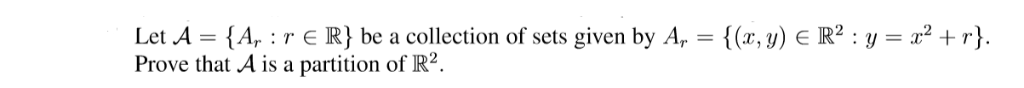{(r, y) E R2 y r} Let A = {A,:r e R} be a collection of sets given by A, = Prove that A is a partition of R2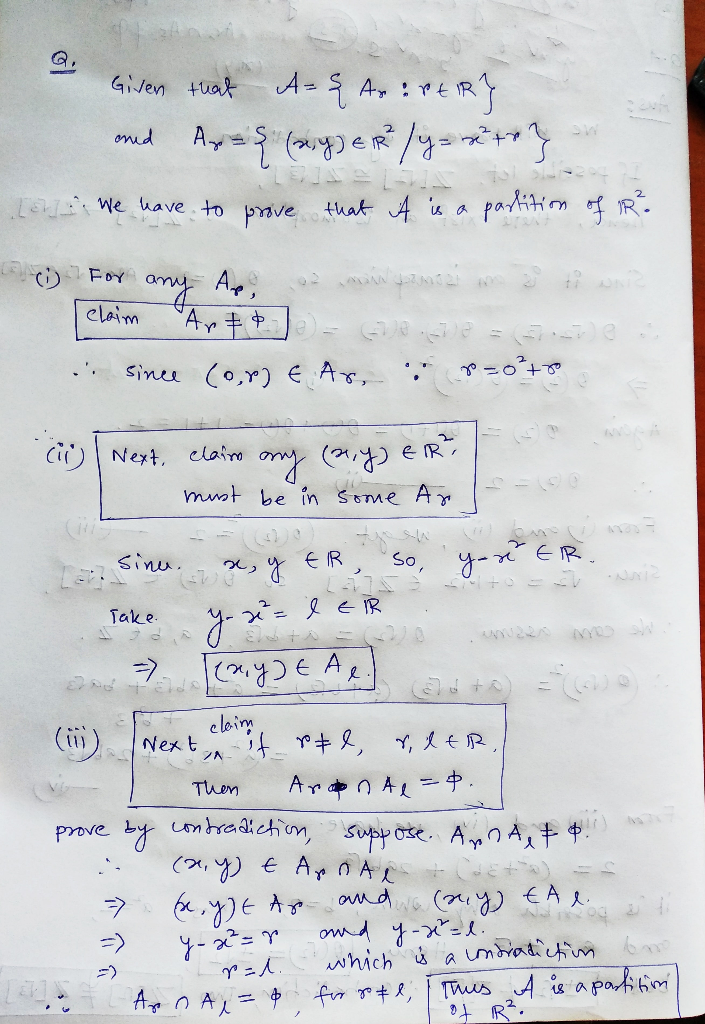##### Add Answer of: {(r, y) E R2 y r} Let A = {A,:r e R} be a collection of sets given by A, = Prove that A is a partition of R2 {(r,...
Similar Homework Help Questions
• ### Let H ⊂ R2 be the line H = {(a, 2a) : a ∈ R}. Consider the collection of all translates of H, i.e...

Let H ⊂ R2 be the line H = {(a, 2a) : a ∈ R}. Consider the collection of all translates of H, i.e., all lines in the plane with slope 2. Find the equivalence relation on R2 defined by this partition of R2 .

• ### Problem 11.9. For two nonempty disjoint sets, and J, let A : E/} be a parti- tion ofR" and Ag' α E J} be a part...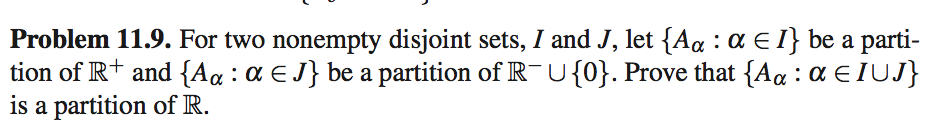Problem 11.9. For two nonempty disjoint sets, and J, let A : E/} be a parti- tion ofR" and Ag' α E J} be a partition of R-u0). Prove that(Aq : α Ε 1w} is a partition of R. Problem 11.9. For two nonempty disjoint sets, and J, let A : E/} be a parti- tion ofR" and Ag' α E J} be a partition of R-u0). Prove that(Aq : α Ε 1w} is a partition of R.

• ### (e) Letf: R2-R2 be given by f(a,y) = (V-y,y) Let A, B be the subsets of...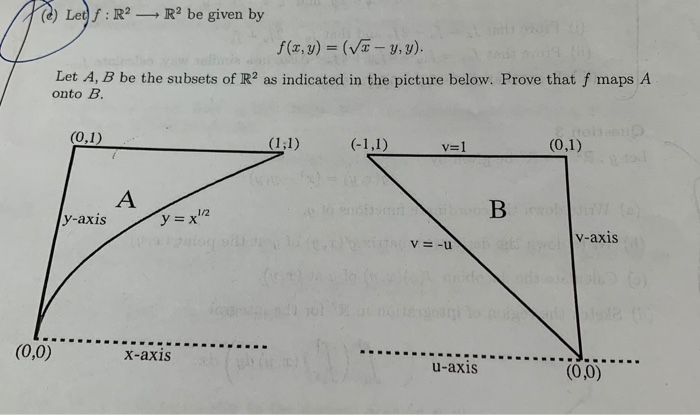(e) Letf: R2-R2 be given by f(a,y) = (V-y,y) Let A, B be the subsets of R2 as indicated in the picture below. Prove that f maps A onto B. (0,1) (1;1) (-1,1) (0,1) v=1 1/2 y-axis y=x2 v-axis v -u b ets t) ide ods.a notteog (0,0) X-axis u-axis (0,0) (e) Letf: R2-R2 be given by f(a,y) = (V-y,y) Let A, B be the subsets of R2 as indicated in the picture below. Prove that f maps A onto...

• ### 1(a) Let f : R2 → R b constant M > 0 such that livf(x,y)|| (0.0)-0. Assume that there exists a e continuously differentiable, with Mv/r2 + уг, for all (z. y) E R2 If(x,y)| 〈 M(x2 + y2)· for all (...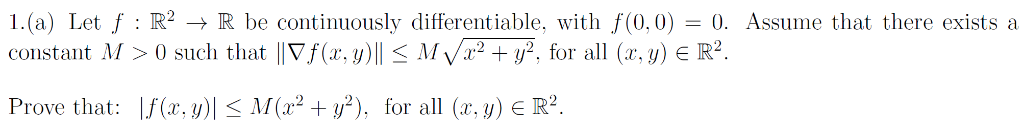1(a) Let f : R2 → R b constant M > 0 such that livf(x,y)|| (0.0)-0. Assume that there exists a e continuously differentiable, with Mv/r2 + уг, for all (z. y) E R2 If(x,y)| 〈 M(x2 + y2)· for all (a·y) E R2 Prove that: 1(a) Let f : R2 → R b constant M > 0 such that livf(x,y)|| (0.0)-0. Assume that there exists a e continuously differentiable, with Mv/r2 + уг, for all (z. y) E R2...

• ### For r ∈R, let Ar = {(x,y,z ∈R^3 . . . x^2 + y^2 −z^2 = r}. Is this a partition of R^3? If so, give a geometric descripti...

For r ∈R, let Ar = {(x,y,z ∈R^3 . . . x^2 + y^2 −z^2 = r}. Is this a partition of R^3? If so, give a geometric description of the partitioning sets (i.e., the equivalence classes of the induced equivalence relation).

• ### Use the law of cosines to prove that isometries preserve angles; that is suppose that T : R2 → R2 is an isometry and let P, Q, R E R2 be three noncollinear points in the plane. Denote the images...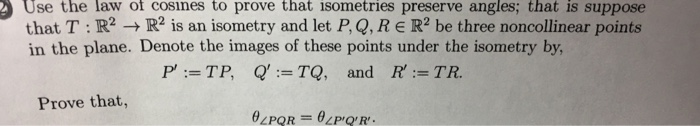Use the law of cosines to prove that isometries preserve angles; that is suppose that T : R2 → R2 is an isometry and let P, Q, R E R2 be three noncollinear points in the plane. Denote the images of these points under the isometry by Q':=TQ, P':=T P, and R :=TR. Prove that, Use the law of cosines to prove that isometries preserve angles; that is suppose that T : R2 → R2 is an isometry and let...

• ### Problem 1: Let F(, y,) be a function given by F(, y, z) (r2+y)e. Let S be the surface in R given ...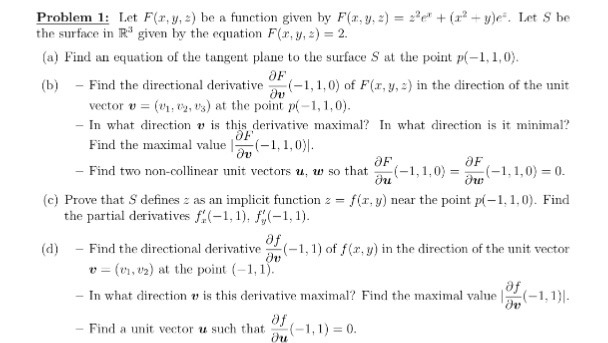Problem 1: Let F(, y,) be a function given by F(, y, z) (r2+y)e. Let S be the surface in R given by the equation Fr, y, 2) 2. (a) Find an equation of the tangent plane to the surface S at the point p(-1,1,0) (b)Find the directional derivative -1,1,0) of F(,y,2) in the direction of the unit vector u = (ui, t», t's) at the point p(-1,1,0) - In what direction is this derivative maximal? In what direction is...

• ### Implicit Function Theorem in Two Variables: Let g: R2 → R be a smooth function. Set {(z, y) E R2 ...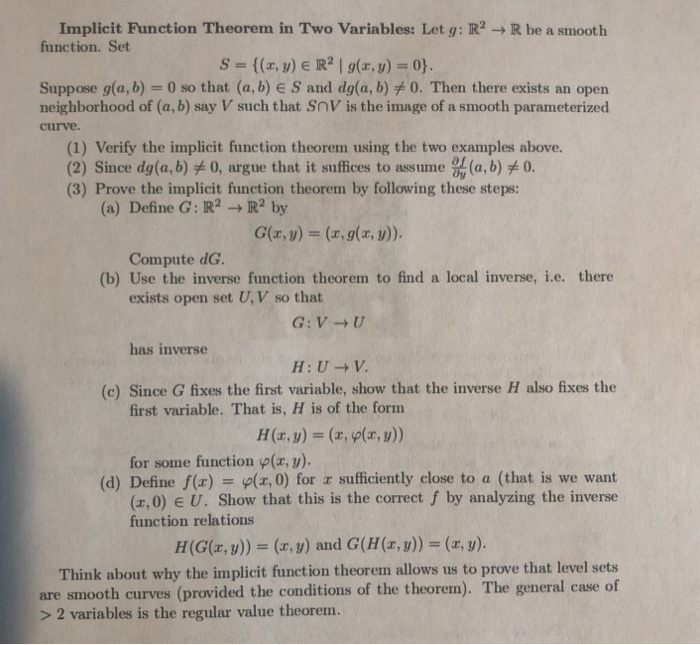Implicit Function Theorem in Two Variables: Let g: R2 → R be a smooth function. Set {(z, y) E R2 | g(z, y) = 0} S Suppose g(a, b)-0 so that (a, b) E S and dg(a, b)メO. Then there exists an open neighborhood of (a, b) say V such that SnV is the image of a smooth parameterized curve. (1) Verify the implicit function theorem using the two examples above. 2) Since dg(a,b) 0, argue that it suffices to...

• ### equivalent 4. Let E C R. Prove that the following statements are (a) E is Lebesgue measurable (b) Given e> 0, the...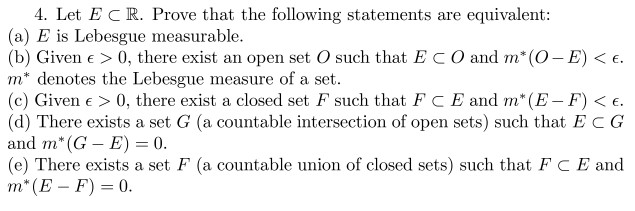equivalent 4. Let E C R. Prove that the following statements are (a) E is Lebesgue measurable (b) Given e> 0, there exist m* denotes the Lebesgue measure of a set (c) Given e 0, there exist a closed set F such that F C E and m* (E- F) < E. (d) There exists a set G (a countable intersection of open sets) such that E C G and m* (G - E) 0 (e) There exists a set...

• ### equivalent 4. Let E C R. Prove that the following statements are (a) E is Lebesgue measurable (b) Given e> 0, the...equivalent 4. Let E C R. Prove that the following statements are (a) E is Lebesgue measurable (b) Given e> 0, there exist m* denotes the Lebesgue measure of a set (c) Given e 0, there exist a closed set F such that F C E and m* (E- F) < E. (d) There exists a set G (a countable intersection of open sets) such that E C G and m* (G - E) 0 (e) There exists a set...

Free Homework App